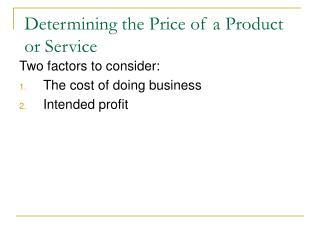DownloadDownload PresentationDetermining the Price of a Product or Service

# Determining the Price of a Product or Service

Télécharger la présentation## Determining the Price of a Product or Service

- - - - - - - - - - - - - - - - - - - - - - - - - - - E N D - - - - - - - - - - - - - - - - - - - - - - - - - - -
##### Presentation Transcript

1. Determining the Price of a Product or Service Two factors to consider: • The cost of doing business • Intended profit

2. Profit: The amount of money left over after all products are paid for and all expenses are covered Price = Cost of Doing Business + Profit

3. Example Expenses \$0.11 Cost \$0.34 \$0.20 Profit Price = \$0.34 + 0.11 + 0.20 = \$0.65

4. 2 Ways to Examine Price After it is Set

5. 1. Markup • The amount of money a business adds to the cost of a product • Expressed as a % • Markup is used to cover all expenses plus make a profit Markup = \$ Amount Store Adds Cost

6. Example Expenses = \$0.11 Profit = \$0.20 Cost = \$0.34 Markup = \$0.11 + 0.20 \$0.34 = 91%

7. Example Therefore, Wal-Mart buys Skittles for \$0.34 and marks them up by 91%

8. 2. Margin • The percentage of the price charged to customers that is not used to pay for the product Margin = \$ Amount Store Adds Selling Price

9. Example Expenses = \$0.11 Profit = \$0.20 Price = \$0.65 Margin = \$0.11 + 0.20 \$0.65 = 48%

10. Example Therefore, every time Wal-Mart sells Skittles it makes a 48% profit margin

11. Markup and Margin Question • Cody needs to make some extra money to buy Christmas presents for all of his ladies so he opens a hotdog stand on Bank St.

12. Markup and Margin Question • He pays \$0.30 for every hotdog that he sells. • Propane and condiments cost him an additional \$0.22 per hotdog. • He wants to make \$0.23 profit per hotdog

13. Markup and Margin Question • What is his selling price? • What is the markup? • What is the margin?

14. Answers Price = Cost of doing business + profit = \$0.30 + 0.22 + 0.23 = \$0.75 Markup = \$ Amount store adds / cost = \$0.22 + 0.23 / 0.30 = \$0.45 / 0.30 = 1.5 OR 150%

15. Answers Margin = \$ Amount store adds / selling price = \$0.22 + 0.23 / \$0.75 = \$0.45 / \$0.75 =0.6 OR 60%

16. Break-Even Analysis • How many units must be sold at a given price to cover all operating costs?

17. Break-Even Analysis Three parts to break-even analysis: • Variable Costs: Costs that depend on the quantity of products or services sold Example:

18. Break-Even Analysis 2. Fixed Costs: Costs that are constant. These do not depend on the quantity of sales. Example:

19. Break-Even Analysis 3. Sometimes called Contribution Margin Example: Gross Profit = Selling Price – Variable Costs

20. Break-Even Analysis • Gross Profit Example • Selling Price = \$1.49 • Variable Cost = \$0.35 • GP = Selling Price - VC • Therefore, \$1.14 of Gross Profit is made with every sale of an Iced Cap • This is used to pay for Fixed Costs

21. Break-Even Point Again, Break-Even Point (BEP) is the # of units that must be sold at a given price to cover all operating costs BEP = Fixed Costs Gross Profit

22. Break-Even Point Back to Cody • He pays \$0.30 for every hotdog • \$0.22 for gas and condiments per hotdog • Sells hotdogs for \$0.75 each • Pays \$200/month to rent the cart (Fixed)

23. What is Cody’s BEP? • Gross Profit = Selling Price – VC = \$0.75 – 0.30 – 0.22 = \$0.23 • BEP = FC / Gross Profit = \$200 / \$0.23 = 870 hotdogs

24. What is Cody’s BEP? • Therefore, Cody has to sell 870 hotdogs in order to cover the cost of renting the cart. • If he doesn’t sell 870 he will lose money!

25. Pricing and BEP Example • Subway has the following costs for a 6”assorted sub it sells: • Bread = \$0.37 • Meat = \$1.68 • Toppings = \$0.39 • Expenses = \$1.30 (includes all other FC and VC) • Subway wants to make \$1.25 per sub

26. What should the price be? • Price = \$4.99

27. What it the markup?What is the margin? • Cost = \$2.44 • Markup = 105% • Margin = 51%

28. What is the Gross Profit? • Assuming that: • Bread = \$0.37 • Meat = \$1.68 • Toppings = \$0.39 • Expenses = \$1.30 (includes all other FC and VC) • Other VC = \$0.90

29. What it the gross profit? • Total VC = \$3.34 • Gross Profit = \$4.99 – 3.34 = \$1.65

30. What is the BEP? • Assume that Subway pays the following monthly Fixed Costs: • Wages \$12,500 • Rent \$2,100 • Hydro \$800

31. What is the BEP? • FC = \$15,400 • BEP = \$15,400 / \$1.65 = 9,333 subs Therefore, every month Subway needs to sell 9,333 subs just to break even!